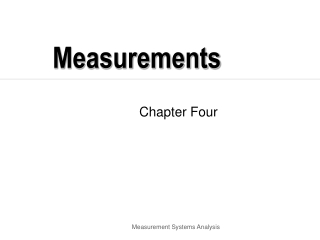DownloadDownload PresentationMeasurements

# Measurements

Télécharger la présentation## Measurements

- - - - - - - - - - - - - - - - - - - - - - - - - - - E N D - - - - - - - - - - - - - - - - - - - - - - - - - - -
##### Presentation Transcript

1. Measurements Chapter Four Measurement Systems Analysis

2. Measurement accuracy and precision Measurement Systems Analysis

3. Accuracy and precision • Measurements are said to be accurate if their tendency is to center around the actual value of the entity being measured. • Measurements are precise if they differ from one another by a small amount. • What can you say about the causes relating to each type? Measurement Systems Analysis

4. Measurement Systems Analysis Measurement Systems Analysis

5. Measured Value Measured Value = ƒ(TV + Ac + Rep + Rpr) TV = true value Ac = gauge accuracy Rep = gauge repeatability Rpr = gauge reproducibility Measurement Systems Analysis

6. Measurement system components • Equipment or gage • Type of gage • Attribute: go-no go, vision systems(part present or not present) • Variable: calipers, probe, coordinate measurement machines • Unit of measurement - usually at least 1/10 of tolerance • Operator and operating instructions Measurement Systems Analysis

7. Measurement error • Measurement error is considered to be the difference between a value measured and the true value. Measurement Systems Analysis

8. Types of gage variation • Systematic variation • Accuracy - improper calibration • Reproducibility - different persons using same equipment with different techniques • Periodic variation • Stability - wear, deterioration, environment • Random variation • Repeatability (unable to locate part to be measured) Measurement Systems Analysis

9. Types of measurement variation • Accuracy • Stability • Reproducibility • Repeatability Measurement Systems Analysis

10. Accuracy Difference between the true average and the observed average. (True average may be obtained by using a more precise measuring tool) Accuracy or mean bias True average Observed average Measurement Systems Analysis

11. Stability The difference in the average of at least 2 sets of measurements obtained with a gage over time. Stability Time 1 Time 2 Measurement Systems Analysis

12. Reproducibility Variation in average of measurements made by different operators using the same gage measuring the same part. True Average Operator C Operator B Operator A Measurement Systems Analysis

13. Repeatability The random variation in measurements when one operator uses the same gage to measure the same part several times. Observed Average True Average Repeatability Measurement Systems Analysis

14. How do we improve gage capability? • Reproducibility • operator training, or • more clearly define measurement scale available to the operator • Repeatability • gage maintenance • gage redesign to better fit application Measurement Systems Analysis